• 循环队列和顺序队列的主要区别在于:循环队列将顺序队列臆造成一个环状空间.在操作上这种异同体现在: 相同点: 在顺序队列和循环队列中,进行出队、入队操作时,队首、队尾指针都要加 1 ,朝前移动。 不同点: 1. 在...
• 循环队列和顺序队列的主要区别在于:循环队列将顺序队列臆造成一个环状空间.在操作上这种异同体现在: 相同点:在顺序队列和循环队列中,进行出队、入队操作时,队首、队尾指针都要加 1 ,朝前移动。 不同点: 1. 在...
前面分析顺序队的时候,我们知道,顺序队存在”假溢出”的问题,这个问题有时会造成很大的内存浪费,循环队列就是为了解决这个问题而提出地一个很巧妙的办法.循环队列和顺序队列的主要区别在于:循环队列将顺序队列臆造成一个环状空间.在操作上这种异同体现在:

相同点:在顺序队列和循环队列中,进行出队、入队操作时,队首、队尾指针都要加 1 ,朝前移动。
不同点:
1. 在循环队列中当队首、队尾指针指向向量上界(MAX_QUEUE_SIZE-1) 时,其加1 操作的结果是指向向量的下界 0 。而在顺序队列中,说明队已满,若此时采用的是动态顺序链,可以增加申请内存.若是采用静态顺序链,只能退出程序.
2. 顺序队列中q.front = q.rear 表示队空,q.rear = MAX_QUEUE_SIZE表示队满.而在循环队列中.front=q.rear表示队空,而无法用.rear=MAX_QUEUE_SIZE表示队满.

判断循环队列队满的两种方法(本文采用第二种方法):

另设一个标志位以区分队列是空还是满
少用一个元素空间,约定以”队列头指针在队列尾指针的下一位置上”,作为队列呈满状态的标志.
第二种方法的实现:
◆ rear 所指的单元始终为空。
◆ 循环队列为空: front=rear 。
◆ 循环队列满: (rear+1)%MAX_QUEUE_SIZE=front 。

循环队列操作及指针变化情况如下图所示: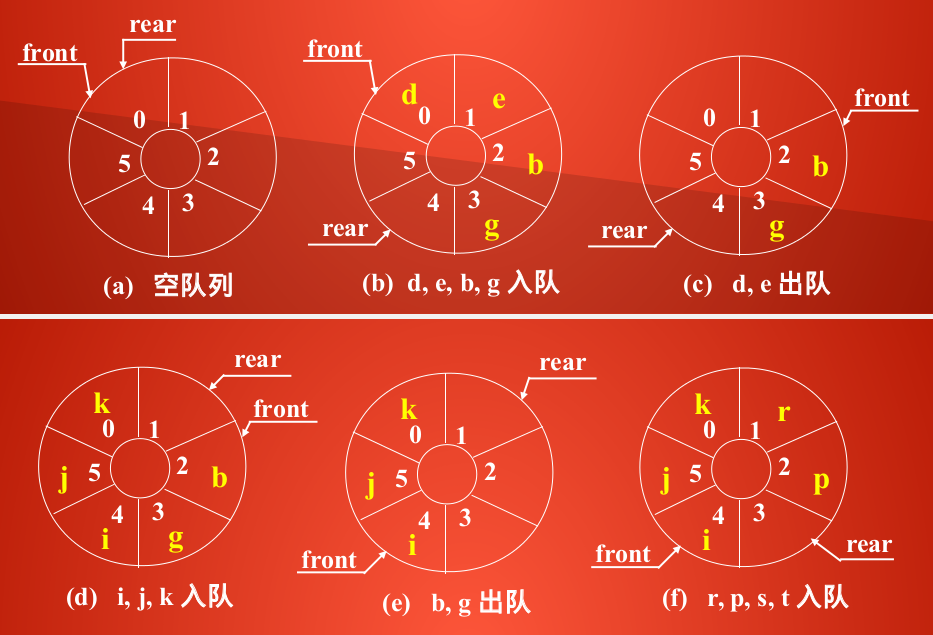循环队列虽然可以解决”假溢出”问题,但是它不能通过动态分配的一维数组来实现,所以在实现循环队列之前,一定要为它设定一个最大队列长度.如果无法预估所需的最大队列长度,只能采用来链表实现.

代码实现:
循环队列和顺序队列的头文件是一样的

/* 循环队列的接口定义头文件 */
#define true 1
#define false 0

/* 队的最大长度 */
#define MAX_QUEUE_SIZE 6
/* 队列的数据类型 */
typedef int datatype;

/* 静态链的数据结构 */
typedef struct queue{
datatype sp_queue_array[MAX_QUEUE_SIZE];
/* 队头 */
int front;
/* 队尾 */
int rear;
}cir_queue;

/* 静态顺序链的接口定义 */

/* 静态链的初始化 */
cir_queue queue_init();

/* 判断队列是否为空,若为空
* 返回true
* 否则返回false
*/
int queue_empty(cir_queue q);

/* 插入元素e为队q的队尾新元素
* 插入成功返回true
* 队满返回false
*/
int queue_en(cir_queue *q, datatype e);

/* 队头元素出队
* 用e返回出队元素,并返回true
* 若队空返回false
*/
int queue_de(cir_queue *q, datatype *e);

/* 清空队 */
void queue_clear(cir_queue *q);

/* 获得队头元素
* 队列非空,用e返回队头元素,并返回true
* 否则返回false
*/
int get_front(cir_queue, datatype *e );

/* 获得队长 */
int queue_len(cir_queue q);

/* 遍历队 */
void queue_traverse(cir_queue q, void(*visit)(cir_queue q));

void visit(cir_queue s);

/* 循环队列的接口实现文件 */
#include<stdio.h>
#include<stdlib.h>
#include"cir_queue.h"

cir_queue queue_init()
{
cir_queue q;
q.front = q. rear  = 0;
return q;
}

int queue_empty(cir_queue q)
{
return q.front == q.rear;
}

int queue_en(cir_queue *q, datatype e)
{
/* 判断队是否已满 */
if (q -> front == (q -> rear + 1) % MAX_QUEUE_SIZE)
return false;
/* 入队 */
q -> sp_queue_array[q -> rear] = e;
q -> rear = (q -> rear + 1) % MAX_QUEUE_SIZE;
return true;
}

int queue_de(cir_queue *q, datatype *e)
{
/* 判断队列是否为空 */
if(q -> front == q -> rear)
return false;
/* 用e返回队头元素 */

*e = q -> sp_queue_array[q -> front];
q -> front = (q -> front + 1 ) % MAX_QUEUE_SIZE;
return true;
}

void queue_clear(cir_queue *q)
{
q -> front = q -> rear = 0;
}

int get_front(cir_queue q, datatype *e)
{
/* 判断队列是否为空 */
if (q.front == q.rear)
return false;
*e = q.sp_queue_array[q.front];
return true;
}

int queue_len(cir_queue q)
{
/* 若front  > rear */
if(q.front > q.rear)
return (q.rear + MAX_QUEUE_SIZE - q.front);
else
return (q.rear - q.front);
}

void queue_traverse(cir_queue q, void(*visit)(cir_queue q))
{
visit(q);
}

void visit(cir_queue q)
{
while(q.front != q.rear)
{
printf("%d ",q.sp_queue_array[q.front]);
q.front = (q.front + 1) % MAX_QUEUE_SIZE;
}
}

int main()
{
cir_queue q = queue_init();
queue_en(&q, 1);
queue_en(&q, 2);
queue_en(&q, 3);
queue_en(&q, 4);
queue_en(&q, 5);
printf("此时队长:length=%d\n", queue_len(q));
queue_traverse(q, visit);
printf("元素6再入队\n");
queue_en(&q, 6);
queue_traverse(q, visit);
datatype *x = (datatype *)malloc(sizeof(*x));
queue_de(&q,x);
printf("出队:%d,此时队长=%d\n", *x, queue_len(q));
printf("元素6再入队\n");
queue_en(&q, 6);
printf("length=%d\n", queue_len(q));
queue_traverse(q,visit);
datatype *e = (datatype *)malloc(sizeof(*e));
queue_de(&q,e);
printf("queue_de(),e=%d length=%d\n", *e,   queue_len(q));
queue_traverse(q, visit);
queue_clear(&q);
queue_traverse(q, visit);
printf("length:%d\n", queue_len(q));
}



运行截图: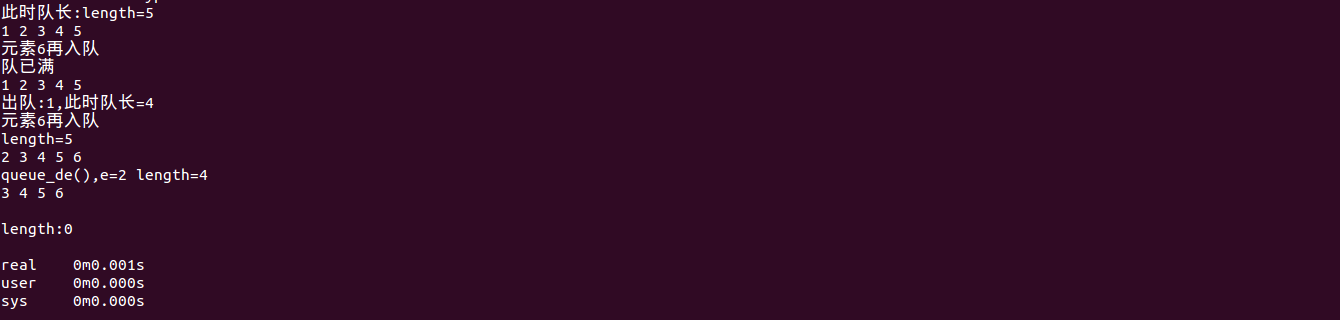展开全文• 循环队列-队列的顺序表示实现循环队列的三种状态循环队列-队列的顺序实现 （代码演示）CycleQueue.hCycleQueue.cppmain.cpp测试结果 循环队列-队列的顺序表示实现 是限制仅在表头删除表尾插入的顺序表。 利用...
 循环队列-队列的顺序表示和实现循环队列的三种状态循环队列-队列的顺序实现 （代码演示）CycleQueue.hCycleQueue.cppmain.cpp测试结果
循环队列-队列的顺序表示和实现
是限制仅在表头删除和表尾插入的顺序表。
利用一组地址连续的存储单元依次存放队列中的数据元素。
因为：队头和队尾的位置是变化的，所以：设头、尾指针。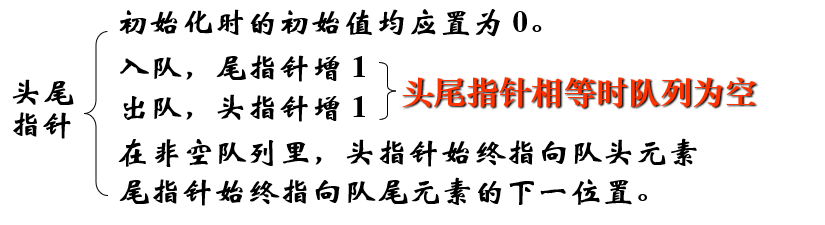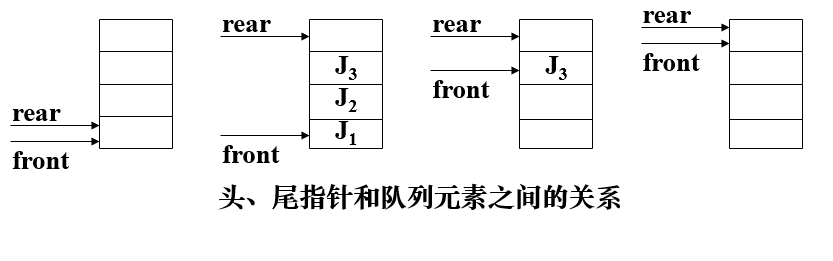在顺序队列中，当尾指针已经指向了队列的最后一个位置的下一位置时，若再有元素入队，就会发生“溢出”。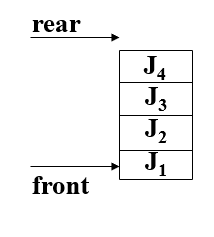“假溢出”——队列的存储空间未满，却发生了溢出。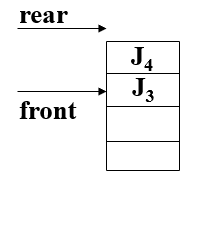解决“假溢出”的问题有两种可行的方法：
(1)、平移元素：把元素平移到队列的首部。效率低。
(2)、将新元素插入到第一个位置上，构成循环队列， 入队和出队仍按“先进先出”的原则进行。 操作效率、空间利用率高。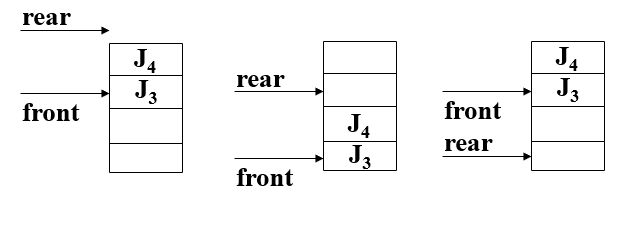循环队列的三种状态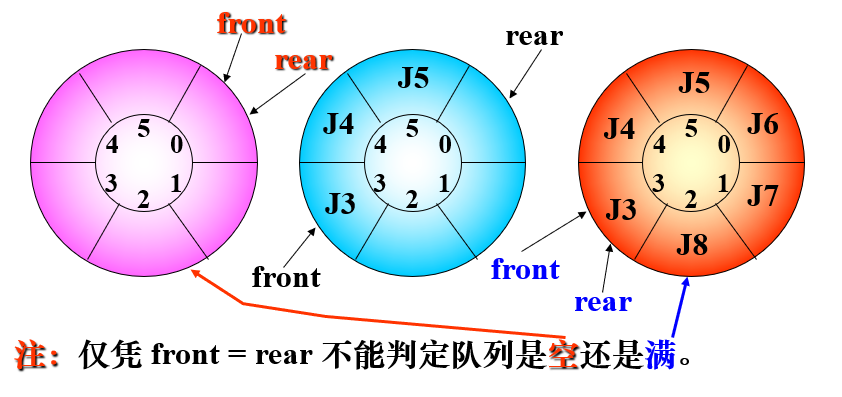解决办法：
(1)、另设一个布尔变量以区别队列的空和满(使用一个计数器记录队列中元素的总数。)；
(2)、少用一个元素的空间，约定入队前测试尾指针在循环意义下加 1 后是否等于头指针，若相等则认为队满；
循环队列-队列的顺序实现 （代码演示）
CycleQueue.h
#pragma once

#define MAXQSIZE  100        //最大队列长度

typedef int QElemType;      //定义数据类型

typedef struct
{
QElemType* base;        // 预分配存储空间基址
int  front;             // 头指针，若队列不空，
// 指向队列头元素
int  rear;              // 尾指针，若队列不空，
// 指向队列尾元素 的下一个位置
} SqQueue;

void InitCycleQueue(SqQueue* Q);//初始化循环队列
void DestroyCycleQueue(SqQueue* Q); //销毁循环队列
int LengthCycleQueue(SqQueue Q); //求循环队列的长度
bool InputCycleQueue(SqQueue* Q, QElemType val); //入循环队列
bool OutputCycleQueue(SqQueue* Q, QElemType * val); //出循环队列
void Show(SqQueue Q); //打印循环队列的元素


CycleQueue.cpp
#include "CycleQueue.h"
#include <malloc.h>
#include <stdlib.h>
#include <stdio.h>

void InitCycleQueue(SqQueue* Q)
{
Q->base = (QElemType*)malloc(MAXQSIZE * sizeof(QElemType));
if (!Q->base)
{
printf("InitQueue file\n");
exit(-1);
}
Q->front = Q->rear = 0;
}

void DestroyCycleQueue(SqQueue* Q) //销毁循环队列
{
free(Q->base);
}

int LengthCycleQueue(SqQueue Q) //求循环队列的长度
{
return (Q.rear - Q.front + MAXQSIZE) % MAXQSIZE;
}

bool InputCycleQueue(SqQueue* Q, QElemType val)//入循环队列
{
if ((Q->rear + 1) % MAXQSIZE == Q->front)
return false;
Q->base[Q->rear] = val;
Q->rear = (Q->rear + 1) % MAXQSIZE;
return true;
}

bool OutputCycleQueue(SqQueue* Q, QElemType* val) //出循环队列
{
if(Q->rear == Q->front)
return false;
*val = Q->base[Q->front];
Q->front = (Q->front + 1) % MAXQSIZE;
return true;
}

void Show(SqQueue Q)//打印循环队列的元素
{
while (Q.front % MAXQSIZE != Q.rear)
printf("%d\t", Q.base[Q.front++]);
printf("\n");
}


main.cpp
#include "CycleQueue.h"
#include <stdio.h>
int main()
{
SqQueue Q;
InitCycleQueue(&Q);
printf("入循环队列:\n");
for (int i = 0; i < 10; i++)
InputCycleQueue(&Q, i * 11);
Show(Q);

QElemType tmp;
printf("出循环队列:\n");
OutputCycleQueue(&Q, &tmp);
Show(Q);

printf("出循环队列:\n");
OutputCycleQueue(&Q, &tmp);
Show(Q);

printf("循环队列长度为%d\n", LengthCycleQueue(Q));

DestroyCycleQueue(&Q);
return 0;
}

测试结果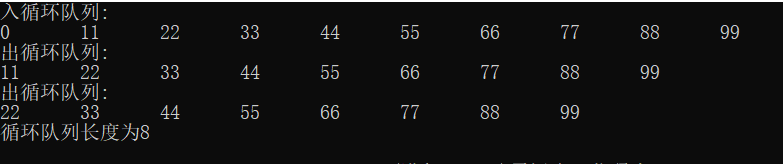展开全文• 栈的先进后出不同，队列的形式是先进先出，队列的想法来自于生活中排队的策略， 顾客在付款结账的时候，按照到来的先后顺序排队结账。先来的顾客先结账，后来的顾客后结账。 队列有两种实现形式：１　顺序表实现...

和栈的先进后出不同，队列的形式是先进先出，队列的想法来自于生活中排队的策略， 顾客在付款结账的时候，按照到来的先后顺序排队结账。先来的顾客先结账，后来的顾客后结账。
队列有两种实现形式：１　顺序表实现　２　循环顺序表
首先来看下顺序表的实现，在python中，队列的实现用list来写十分的方便。实现方式如下：
class line_queue():
def __init__(self):
self._elem=[]
def push(self,elem):
self._elem.append(elem)
def pop(self):
elem=self._elem.pop(0)
return elem
def queue_length(self):
return len(self._elem)
和栈唯一的区别是，这里pop是pop(0),也就是首先进队列的数据出列。这个实现很简单，但是有一个问题，每次有元素出队列的时候，元素都必须要进行前移。这就带来了一个问题，它的操作复杂度为Ｏ(n)，而不是O(1)。只有从尾部弹出元素也就是先进后出的时候复杂度为O(1).
那么如何才能满足Ｏ(1)的出列复杂度呢。我们可以考虑记住队头和队尾的位置。每次出队的时候直接将队头位置的元素弹出就可以了。具体的实现可以参考下图下面来看下代码的实现：
class line_queue_update():
def __init__(self):
self._elem=[]
def push(self,elem):
self._elem.append(elem)
self.rear+=1
def pop(self):
return elem
def queue_length(self):
return len(self._elem)
def get_elem(self):
print self._elem

if __name__=="__main__":
q=line_queue_update()
for i in range(10):
q.push(i)
print 'The length is %d' % q.queue_length()
q.pop()
q.pop()
q.push(90)
q.push(100)
q.push(200)
print 'The length is %d' % q.queue_length()
运行结果如下：
/usr/bin/python2.7 /home/zhf/py_prj/data_struct/chapter5.py
The length is 10
The length is 13
这个方法的实现出队列的复杂度就是Ｏ(1)。但是我们同时也注意到另外一个问题，那就是尽管我们曾经pop元素。但是整个list的长度在不断的增加。这是为什么呢，是因为队头/对尾位置变量的值将随着操作移动。从操作效率来看，每个操作都在Ｏ(1)时间完成。但另一方面，表中元素序列随着操作向表尾方向移动，这就导致了在表的前端留下来了越来越多的空间，这些空间就是那些已经出队的元素曾经占据的空间。在c语言中，表元素存储的大小是固定的，经过反复的入队和出队操作。一定会在某次入队时出现队尾溢出也就是表满的情况。对于python而言，由于list是自动增长的，随着操作进行，表前端留下了越来越大的空区，而且这片空区永远也不会用到，完全浪费了。
前面介绍了顺序队列的两种实现方式，第一种时间效率无法满足，空间效率满足。　第二种时间效率满足，空间效率无法满足。两种策略都有各自的缺点，那么是否有一种方式既能满足时间效率也能满足空间效率呢。这就需要用到队列的第二种实现方式：循环顺序表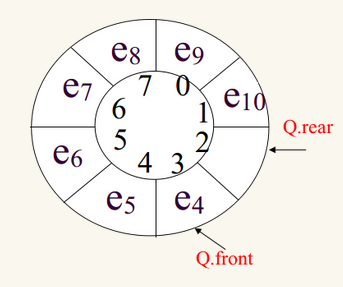那么在这个结构里面，数据就保存在q.front到q.rear的位置里面。两个变量的差就是队列里面的元素个数。这种结构下元素始终在一个环里进行轮转。空位置可以被新插入的元素占据。
那么在环形结构中，需要注意以下３点：
１当队列为空或者为满的时候，q.front=q.rear。由于q.front=q.rear对应队空和队满两种情况，因此为了区分，可以通过队列长度来判断，当q.front=q.rear且q.len=q.max的时候认为为队满，否则为队空。
2 出队的时候q.front=(q.front+1)%q.len
3 入队的时候q.rear=(q.rear+1)%q.len
第２点和第３点的实现方式和顺序表不一样，在更新队头和队尾位置的时候需要进行求余操作，这是因为循环队列的空间大小固定，队头和队尾的位置是相对增长的，不是绝对增长的。下面来看下具体的实现代码
class squeue():
def __init__(self,init_len=8):
self._len=init_len
self._elem=*init_len
self._rear=0
self._num=0
def is_empty(self):
return self._num == 0
def is_full(self):
return self._num == self._len
def dequeue(self):
if self.is_empty():
raise BaseException('queue is empty')
self._num-=1
return e

def enqueue(self,elem):
if self.is_full():
raise BaseException('queue is full')
self._elem[self._rear] = elem
self._rear=(self._rear+1) % self._len
self._num+=1
def get_elem(self):
print self._elem

if __name__=="__main__":
q=squeue()
for i in range(8):
q.enqueue(i)
q.get_elem()
q.dequeue()
q.enqueue(100)
q.get_elem()
首先插入８个元素，然后出队一个元素
/usr/bin/python2.7 /home/zhf/py_prj/data_struct/chapter5.py
[0, 1, 2, 3, 4, 5, 6, 7]
[100, 1, 2, 3, 4, 5, 6, 7]
当继续插入元素的时候，q.enqueue(100)会提示队列已满
/usr/bin/python2.7 /home/zhf/py_prj/data_struct/chapter5.py
Traceback (most recent call last):
File "/home/zhf/py_prj/data_struct/chapter5.py", line 196, in <module>
q.enqueue(100)
File "/home/zhf/py_prj/data_struct/chapter5.py", line 173, in enqueue
raise BaseException('queue is full')
BaseException: queue is full
我们可以添加一个扩充队列的操作，防止队列满导致插入失败
def expand(self):
old_len=self._len
self._len*=2
new_elems=*self._len
for i in range(old_len):
if __name__=="__main__":
q=squeue()
for i in range(8):
q.enqueue(i)
q.get_elem()
q.dequeue()
q.enqueue(100)
q.get_elem()
q.enqueue(200)
q.get_elem()
运行结果如下：
/usr/bin/python2.7 /home/zhf/py_prj/data_struct/chapter5.py
[0, 1, 2, 3, 4, 5, 6, 7]
[100, 1, 2, 3, 4, 5, 6, 7]
[100, 1, 2, 3, 4, 5, 6, 7, 0, 200, 0, 0, 0, 0, 0, 0]

转载于:https://www.cnblogs.com/zhanghongfeng/p/8469904.html
展开全文• C语言实现链式队列、顺序队列和循环队列 队列 队列是一种特殊的线性表，特殊之处在于它只允许在表的前端（front）进行删除操作，而在表的后端（rear）进行插入操作，和栈一样，队列是一种操作受限制的线性表。...
C语言实现链式队列、顺序队列和循环队列
队列

队列是一种特殊的线性表，特殊之处在于它只允许在表的前端（front）进行删除操作，而在表的后端（rear）进行插入操作，和栈一样，队列是一种操作受限制的线性表。进行插入操作的端称为队尾，进行删除操作的端称为队头。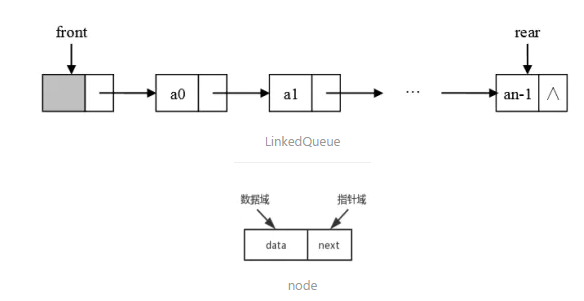循环队列

循环队列就是将队列存储空间的最后一个位置绕到第一个位置,形成逻辑上的环状空间,供队列循环使用。在循环队列结构中,当存储空间的最后一个位置已被使用而再要进入队运算时,只需要存储空间的第一个位置空闲,便可将元素加入到第一个位置,即将存储空间的第一个位置作为队尾。

链式队列
链式队列是一种特殊的线性链表，这个链表你只能从表尾插入，从表头删除。

顺序队列
顺序队列是队列的顺序存储结构，顺序队列实际上是运算受限的顺序表。

common.h
#ifndef _COMMON_H_
#define _COMMON_H_

#include<stdio.h>
#include<assert.h>
#include<stdbool.h>
#include<stdlib.h>

#define ElemType int

void Swap(ElemType *a, ElemType *b)
{
ElemType tmp = *a;
*a = *b;
*b = tmp;
}

#endif /* _COMMON_H_ */


queue.h
#ifndef _QUEUE_H_
#define _QUEUE_H_

#include"common.h"
///
//顺序队列

#define SEQ_QUEUE_DEFAULT_SIZE 8

typedef struct SeqQueue
{
ElemType *base;
int       capacity;
int       front;
int       rear;
}SeqQueue;

void SeqQueueInit(SeqQueue *psq);
bool SeqQueueIsFull(SeqQueue *psq);
bool SeqQueueIsEmpty(SeqQueue *psq);
void SeqQueueEnque(SeqQueue *psq, ElemType x);
void SeqQueueDeque(SeqQueue *psq);
ElemType SeqQueueFront(SeqQueue *psq);
void SeqQueuePrint(SeqQueue *psq);

void SeqQueueInit(SeqQueue *psq)
{
assert(psq != NULL);
psq->base = (ElemType*)malloc(sizeof(ElemType) * SEQ_QUEUE_DEFAULT_SIZE);
assert(psq->base != NULL);
psq->capacity = SEQ_QUEUE_DEFAULT_SIZE;
psq->front = psq->rear = 0;
}

bool SeqQueueIsFull(SeqQueue *psq)
{
assert(psq != NULL);
return psq->rear >= psq->capacity;
}
bool SeqQueueIsEmpty(SeqQueue *psq)
{
assert(psq != NULL);
return psq->front == psq->rear;
}
void SeqQueueEnque(SeqQueue *psq, ElemType x)
{
assert(psq != NULL);
if (SeqQueueIsFull(psq))
{
printf("队列已满, %d 不能入队.\n", x);
return;
}
psq->base[psq->rear++] = x;
}
void SeqQueueDeque(SeqQueue *psq)
{
assert(psq != NULL);
if (SeqQueueIsEmpty(psq))
{
printf("队列已空，不能出队.\n");
return;
}

psq->front++;
}
ElemType SeqQueueFront(SeqQueue *psq)
{
assert(psq != NULL);
if (SeqQueueIsEmpty(psq))
{
printf("队列已空，不能取对头元素.\n");
return;
}

return psq->base[psq->front];
}
void SeqQueuePrint(SeqQueue *psq)
{
assert(psq != NULL);
for (int i = psq->front; i < psq->rear; ++i)
printf("%d ", psq->base[i]);
printf("\n");
}

///
//循环队列
#define CIRCLE_QUEUE_DEFAULT_SIZE 8
#define CIRCLE_QUEUE_INC_SIZE 4

typedef struct CircleQueue
{
ElemType *base;
int       capacity;
int       front;
int       rear;
}CircleQueue;

void CircleQueueInit(CircleQueue *psq);
bool CircleQueueIsFull(CircleQueue *psq);
bool CircleQueueIsEmpty(CircleQueue *psq);
void CircleQueueEnque(CircleQueue *psq, ElemType x);
void CircleQueueDeque(CircleQueue *psq);
ElemType CircleQueueFront(CircleQueue *psq);
void CircleQueuePrint(CircleQueue *psq);

void CircleQueueInit(CircleQueue *psq)
{
assert(psq != NULL);
psq->base = (ElemType*)malloc(sizeof(ElemType) * (CIRCLE_QUEUE_DEFAULT_SIZE + 1));
assert(psq->base != NULL);
psq->capacity = SEQ_QUEUE_DEFAULT_SIZE + 1;
psq->front = psq->rear = 0;
}
bool CircleQueueIsFull(CircleQueue *psq)
{
assert(psq != NULL);
return ((psq->rear + 1) % psq->capacity) == psq->front;
}
bool CircleQueueIsEmpty(CircleQueue *psq)
{
assert(psq != NULL);
return psq->front == psq->rear;
}
void CircleQueueEnque(CircleQueue *psq, ElemType x)
{
assert(psq != NULL);
if (CircleQueueIsFull(psq))
{
printf("循环队列已满, %d 不能入队.\n", x);
return;
}
psq->base[psq->rear] = x;
psq->rear = (psq->rear + 1) % psq->capacity;
}
void CircleQueueDeque(CircleQueue *psq)
{
assert(psq != NULL);
if (CircleQueueIsEmpty(psq))
{
printf("循环队列已空，不能出队.\n");
return;
}
psq->front = (psq->front + 1) % psq->capacity;
}
ElemType CircleQueueFront(CircleQueue *psq)
{
assert(psq != NULL);
if (CircleQueueIsEmpty(psq))
{
printf("循环队列已空，不能取对头元素.\n");
return;
}

return psq->base[psq->front];
}
void CircleQueuePrint(CircleQueue *psq)
{
assert(psq != NULL);
for (int i = psq->front; i != psq->rear;)
{
printf("%d ", psq->base[i]);
i = (i + 1) % psq->capacity;
}
printf("\n");
}
///
//链式队列

//#undef ElemType

{
ElemType data;

{

{
assert(pq != NULL);
pq->front = pq->rear = NULL;
}

{
assert(pq != NULL);
assert(node != NULL);
node->data = x;

if (pq->front == NULL)
pq->front = pq->rear = node;
else
{
pq->rear = node;
}
}
{
assert(pq != NULL);
if (pq->front != NULL)
{
free(p);
}
}
{
assert(pq != NULL);
while (p != NULL)
{
printf("%d ", p->data);
}
printf("\n");
}

{
return pq->front == NULL;
}

{
assert(pq->front != NULL);
return pq->front->data;
}

#endif /* _QUEUE_H_ */

SeqQueueMain.c
#define _CRT_SECURE_NO_WARNINGS 1
#include "queue.h"
void main()
{
SeqQueue Q;
SeqQueueInit(&Q);
SeqQueueEnque(&Q, 1);
SeqQueueEnque(&Q, 2);
SeqQueueEnque(&Q, 3);
SeqQueueEnque(&Q, 4);
SeqQueueEnque(&Q, 5);
SeqQueueEnque(&Q, 6);
SeqQueueEnque(&Q, 7);
SeqQueueEnque(&Q, 8);
SeqQueueEnque(&Q, 9);
SeqQueuePrint(&Q);
printf("队头 = %d\n", SeqQueueFront(&Q));
SeqQueueDeque(&Q);
SeqQueuePrint(&Q);
SeqQueueDeque(&Q);
SeqQueuePrint(&Q);
printf("队头 = %d\n", SeqQueueFront(&Q));
system("pause");
}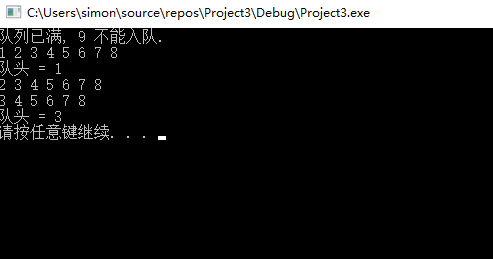#define _CRT_SECURE_NO_WARNINGS 1
#include "queue.h"
void main()
{
printf("出队:%d\n", val);
system("pause");
}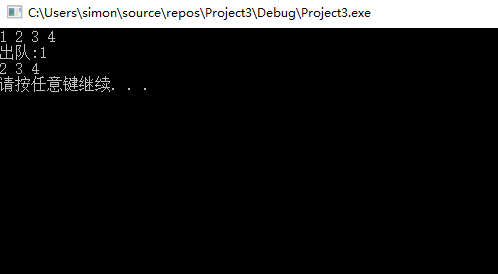CircleQueueMain.h
#define _CRT_SECURE_NO_WARNINGS 1
#include "queue.h"
void main()
{
CircleQueue Q;
CircleQueueInit(&Q);
CircleQueueEnque(&Q, 1);
CircleQueueEnque(&Q, 2);
CircleQueueEnque(&Q, 3);
CircleQueueEnque(&Q, 4);
CircleQueueEnque(&Q, 5);
CircleQueueEnque(&Q, 6);
CircleQueueEnque(&Q, 7);
CircleQueueEnque(&Q, 8);
CircleQueuePrint(&Q);
printf("队头 = %d\n", CircleQueueFront(&Q));
CircleQueueDeque(&Q);
CircleQueueEnque(&Q, 9);
CircleQueuePrint(&Q);
printf("队头 = %d\n", CircleQueueFront(&Q));

system("pause");
}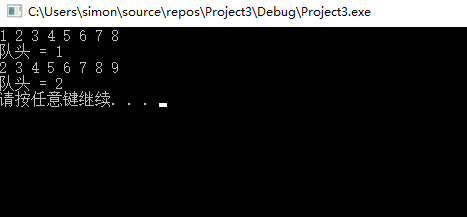展开全文数据结构 算法
• 队列是队尾添加元素，队头删除元素，先进先出...那为什么这个下面的代码可以适用于非循环队列和循环队列呢，原因就在于判断队列为空和满的条件，即当当(rear+1) % MAXSIZE = front时为满这个条件，下面分析一下这个条件
• 队列和循环队列的唯一区别就是，队列的front和rear对应的元素下标被影射成front%maxSize，rear%maxSize。/***循环队列*@param泛型T*/publicclassCircleSequenceQueue{privateT[]data;privateintfront;privateintrear...
• 队列和循环队列的唯一区别就是，队列的front和rear对应的元素下标被影射成front%maxSize，rear%maxSize。/***循环队列*@param泛型T*/publicclassCircleSequenceQueue{privateT[]data;privateintfront;privateintrear...
• 循环队列 #include<iostream> #define MAXIMUN 1000 using namespace std; typedef struct queue_node //循环队列 { int* data; //记录队列的元素个数 int front,rear,count; //记录队头队尾元素...
• 2、循环队列：将顺序队列臆造为一个环状的空间 性质 队空条件：front == rear 队满条件：(rear+1) %QueueSize == front 队列长度：(rear—front + QueueSize) % QueueSize （1）队列初始化时，frontrear值都为...数据结构
• 一、队列的概念：　队列（简称作队，Queue）也是一种特殊的线性表，队列的数据元素以及数据元素间的逻辑关系线性表完全相同，其差别是线性表允许在任意位置插入删除，而队列只允许在其一端进行插入操作在其另...
• 循环队列和顺序队列  队列的存储实现方式有哪些？ 顺序存储（数组）和链式存储（链表），此博文描述的是数组的实现（后续更新链表实现）  代码实现 初始化队列：初始化一个size长度的队列，队列的值都为0 判断队列...
• //队列的顺序表示实现——循环队列循环队列是队列的顺序表示） //自学中，加油！！！ #include<iostream> using namespace std; const int MaxQSize=5; #define QElemType double typedef struct { ...数据结构 算法
• 队列的顺序存储结构（循环队列）#define MAX_QSIZE 5//最大队列长度+1struct SqQueue { QElemType *base; int front;//头指针，若队列不空，指向队列头元素 int rear;//尾指针，若队列不空，指向队列尾元素的下一...c 数据结构 计算机专业
• 循环队列-队列的顺序表示实现使用一组地址连续的存储单元来保存队列元素，并使用两个变量分别指向队列的前端尾端#include&lt;stdio.h&gt; #define MAXNUM 100 #define OK 1 #define ERROR 0 #define ...
• // -----循环队列一队列 的顺序存储结构----- # define MAXQSIZE 100 //最大队列长度 typedef struct { int *base; //初始化的动态分配存储空间 int front ; //头指针,若队列不空,指向队列头元素 int rear ; //尾...
• 对于循环队列，也就是队列的顺序存储结构，面临着数组可能溢出的问题，所以还需要研究一下不需要担心队列长度的链式存储结构。循环队列的插入删除时间复杂度都不高都是O(1)，不需要移动队列里面的元素。 #...算法 C
• 队列的定义 队列是只允许在一端进行插入操作，而在另一端进行删除操作的线性表 队列是一种先进先出（First In Last Out）的线性表，简称FIFO 允许插入的一端称为队尾，允许删除的一端称为队头 队列接口Queue的定义...
• 队列也有两种表示形式，顺序和链式。 与顺序栈相类似，在队列顺序存储结构中，除了用一组地址连续的存储单元依次存放从队列头到队列尾的元素之外，尚需附设两个整形变量frontrear分别指示队列头元素及队列尾...
• typedef struct//构造循环队列数据类型 { int *base;//队列数组指针 int front; int rear; }CircleQueue; void InitQueue(CircleQueue &Q);//初始化创造循环队表 void QueueLength(CircleQueue Q);/数据结构 algorithm
• 由于稀疏矩阵中存在大量的“空”值，占据了大量的存储空间，而真正有用的数据却少之又少， 且在计算时浪费资源，所以要进行压缩存储以节省存储空间计算方便。 举例说明 右边稀疏数组的翻译： 第1行：二维数组...java 数据结构 算法...